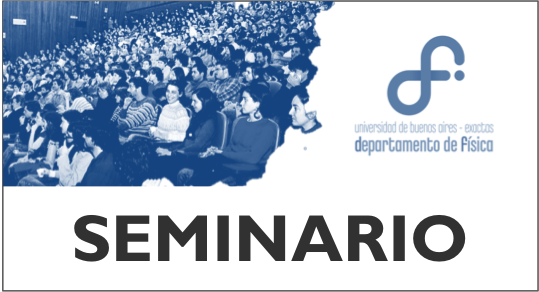## Seminario Conjunto - A. Abedi - E. KhosraviEn el Aula Seminario, 2do piso, Pab. I,

Lunes 18/12, 14hs:

1. Correlated electron-nuclear dynamics
ALI ABEDI

2. The exact potential driving the electron dynamics in enhanced ionization of H2+- isotopes effects
ELHAM KHOSRAVI

Correlated Electron-Nuclear Dynamics

The coupling between electronic and nuclear motion plays an important role in many fascinating phenomena, such as superconductivity, the process of vision, as well as photo-synthesis. There are standard approximations such as Ehrenfest dynamics or surface hopping that partially capture the non-adiabatic effects. As a first step towards a full ab-initio treatment of the coupled electron-nuclear system, we deduce an exact factorization of the complete wavefunction into a purely nuclear part and a many-electron wavefunction which parametrically depends on the nuclear configuration. We derive formally exact equations of motion for the nuclear and electronic wavefunctions [1-3]. These exact equations lead to a rigorous definition of time-dependent potential energy surfaces (TDPES) as well as time-dependent geometric phases. We analyze features of the TDPES in two topically demanding situations:
molecules in strong fields [1-3] and splitting of a nuclear wave-packet at avoided crossings  Born-Oppenheimer potential energy surfaces. In addition, by studying a numerically exactly solvable model we demonstrate that the molecular Berry phase and the corresponding non-analyticity in the electronic Born-Oppenheimer wavefunction is, in general, not a true topological feature of the exact solution of the full electron-nuclear Schroedinger equation and only appear in the limit of infinite nuclear mass .

Finally, we present a novel mixed quantum-classical approach  to the coupled electron-nuclear dynamics based on the equations of motion for the electronic and nuclear subsystems within the exact factorization framework. The nuclear equation is a standard Schroedinger equation containing a TDPES as well as a time-dependent vector potential. Starting from these equations, the correct classical limit of the nuclear dynamics is worked out by taking the classical limit of the exact time-dependent Schroedinger equation satisfied by the nuclear wave function. The effect of the time-dependent scalar and vector potentials, representing the exact electronic back-reaction on the nuclear subsystem, is consistently derived within the classical approximation. Using a model system, we examine the performance of the proposed mixed quantum-classical scheme in comparison with exact calculations, in the presence of strong non-adiabatic coupling between the electronic and nuclear motion.

References:
 A. Abedi, N. T. Maitra, and E. K. U. Gross, Physical Review Letters 105 123002 (2010).
 A. Abedi, N. T. Maitra, and E. K. U. Gross, Journal of Chemical Physics 137 22A530 (2012)
 A. Abedi, F. Agostini, Y. Suzuki, E. K. U. Gross, Physical Review Letters 110 263001 (2013).
 S. K. Min, A. Abedi, K. S. Kim, and E. K. U. Gross, Physical Review Letters 113 263004 (2014).
 A. Abedi, F. Agostini, and E. K. U. Gross, Europhysics Letters 106 33001 (2014).

The exact potential driving the electron dynamics in enhanced ionization of H2+- isotopes effects

The exact potential  driving the electron's dynamics  in enhanced
ionization of H2+ can have large contributions arising from dynamical electron-
nuclear correlation, going beyond what any Coulombic-based model can
provide . This potential is defined via the exact factorization of the molecular
wavefunction that allows the construction of a Schrödinger equation for the
electronic system, in which the potential contains exactly the effect of coupling
to the nuclear system and any external fields [1,2]. To investigate nuclear-mass-
dependence in enhanced ionization, we study isotopologues of H2+ . We
decompose the exact potential into components that naturally arise from the
conditional wavefunction, and also into components arising from the marginal
electronic wavefunction, and compare the performance of propagation on these
different components as well as approximate potentials based on the quasi-
static or Hartree approximation with the exact propagation .

References:
. A. Abedi, N. T. Maitra, and E. K. U. Gross, Phys. Rev. Lett. 105, 123002 (2010).
. Y. Suzuki, A. Abedi, N. T. Maitra, K. Yamashita, and E. K. U. Gross, Phys. Rev. A 89, 040501 (2014).
. E. Khosravi, A. Abedi, and N. T. Maitra, Phys. Rev. Lett. 115, 263002 (2015)
. E. Khosravi, A. Abedi, A. Rubio, N. T. Maitra, PCCP, 19 , 8269 (2017)